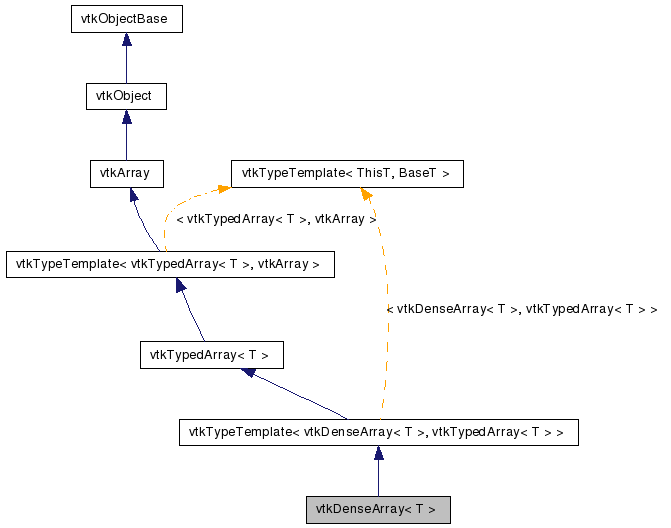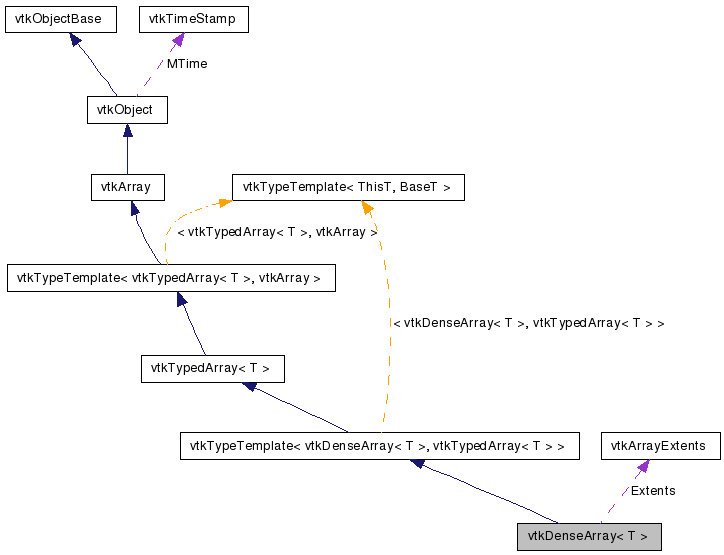vtkDenseArray< T > Class Template Reference

#include <vtkDenseArray.h>

Inheritance diagram for vtkDenseArray< T >:[legend]
Collaboration diagram for vtkDenseArray< T >:[legend]

Detailed Description

template<typename T> class vtkDenseArray< T >

Contiguous storage for N-way arrays.

vtkDenseArray is a concrete vtkArray implementation that stores values using a contiguous block of memory. Values are stored with fortran ordering, meaning that if you iterated over the memory block, the left-most coordinates would vary the fastest.

In addition to the retrieval and update methods provided by vtkTypedArray, vtkDenseArray provides methods to:

Fill the entire array with a specific value.

Retrieve a pointer to the storage memory block.

vtkArray, vtkTypedArray, vtkSparseArray
Thanks:
Tests:
vtkDenseArray (Tests)

Definition at line 57 of file vtkDenseArray.h.

Public Member Functions

void PrintSelf (ostream &os, vtkIndent indent)
vtkArrayExtents GetExtents ()
vtkIdType GetNonNullSize ()
void GetCoordinatesN (const vtkIdType n, vtkArrayCoordinates &coordinates)
vtkArrayDeepCopy ()
const T & GetValue (const vtkArrayCoordinates &coordinates)
const T & GetValueN (const vtkIdType n)
void SetValue (const vtkArrayCoordinates &coordinates, const T &value)
void SetValueN (const vtkIdType n, const T &value)
void Fill (const T &value)
T & operator[] (const vtkArrayCoordinates &coordinates)
const T * GetStorage () const
T * GetStorage ()

Static Public Member Functions

static vtkDenseArray< T > * New ()

Protected Member Functions

vtkDenseArray ()
~vtkDenseArray ()

Constructor & Destructor Documentation

template<typename T>
 vtkDenseArray< T >::vtkDenseArray ( ) [protected]

template<typename T>
 vtkDenseArray< T >::~vtkDenseArray ( ) [protected]

Member Function Documentation

template<typename T>
 static vtkDenseArray* vtkDenseArray< T >::New ( ) [static]

Create an object with Debug turned off, modified time initialized to zero, and reference counting on.

Reimplemented from vtkObject.

template<typename T>
 void vtkDenseArray< T >::PrintSelf ( ostream & os, vtkIndent indent ) [virtual]

Methods invoked by print to print information about the object including superclasses. Typically not called by the user (use Print() instead) but used in the hierarchical print process to combine the output of several classes.

Reimplemented from vtkTypedArray< T >.

template<typename T>
 vtkArrayExtents vtkDenseArray< T >::GetExtents ( ) [virtual]

Returns the extents (the number of dimensions and size along each dimension) of the array.

Implements vtkArray.

template<typename T>
 vtkIdType vtkDenseArray< T >::GetNonNullSize ( ) [virtual]

Returns the number of non-null values stored in the array. Note that this value will equal GetSize() for dense arrays, and will be less-than-or-equal to GetSize() for sparse arrays.

Implements vtkArray.

template<typename T>
 void vtkDenseArray< T >::GetCoordinatesN ( const vtkIdType n, vtkArrayCoordinates & coordinates ) [virtual]

Returns the coordinates of the n-th value in the array, where n is in the range [0, GetNonNullSize()). Note that the order in which coordinates are visited is undefined, but is guaranteed to match the order in which values are visited using vtkTypedArray::GetValueN() and vtkTypedArray::SetValueN().

Implements vtkArray.

template<typename T>
 vtkArray* vtkDenseArray< T >::DeepCopy ( ) [virtual]

Returns a new array that is a deep copy of this array.

Implements vtkArray.

template<typename T>
 const T& vtkDenseArray< T >::GetValue ( const vtkArrayCoordinates & coordinates ) [virtual]

Returns the value stored in the array at the given coordinates. Note that the number of dimensions in the supplied coordinates must match the number of dimensions in the array.

Implements vtkTypedArray< T >.

template<typename T>
 const T& vtkDenseArray< T >::GetValueN ( const vtkIdType n ) [virtual]

Returns the n-th value stored in the array, where n is in the range [0, GetNonNullSize()). This is useful for efficiently visiting every value in the array. Note that the order in which values are visited is undefined, but is guaranteed to match the order used by vtkArray::GetCoordinatesN().

Implements vtkTypedArray< T >.

template<typename T>
 void vtkDenseArray< T >::SetValue ( const vtkArrayCoordinates & coordinates, const T & value ) [virtual]

Overwrites the value stored in the array at the given coordinates. Note that the number of dimensions in the supplied coordinates must match the number of dimensions in the array.

Implements vtkTypedArray< T >.

template<typename T>
 void vtkDenseArray< T >::SetValueN ( const vtkIdType n, const T & value ) [virtual]

Overwrites the n-th value stored in the array, where n is in the range [0, GetNonNullSize()). This is useful for efficiently visiting every value in the array. Note that the order in which values are visited is undefined, but is guaranteed to match the order used by vtkArray::GetCoordinatesN().

Implements vtkTypedArray< T >.

template<typename T>
 void vtkDenseArray< T >::Fill ( const T & value )

Fills every element in the array with the given value.

template<typename T>
 T& vtkDenseArray< T >::operator[] ( const vtkArrayCoordinates & coordinates )

Returns a value by-reference, which is useful for performance and code-clarity.

template<typename T>
 const T* vtkDenseArray< T >::GetStorage ( ) const

Returns a read-only reference to the underlying storage. Values are stored contiguously with fortran ordering.

template<typename T>
 T* vtkDenseArray< T >::GetStorage ( )

Returns a mutable reference to the underlying storage. Values are stored contiguously with fortran ordering. Use at your own risk!

The documentation for this class was generated from the following file:

Generated on Wed Jun 3 18:59:57 2009 for VTK by1.5.6